Thank you for visiting nature.com. You are using a browser version with limited support for CSS. To obtain the best experience, we recommend you use a more up to date browser (or turn off compatibility mode in Internet Explorer). In the meantime, to ensure continued support, we are displaying the site without styles and JavaScript.

# Experimental violation of Bell inequalities for multi-dimensional systems

## Abstract

Quantum correlations between spatially separated parts of a d-dimensional bipartite system (d ≥ 2) have no classical analog. Such correlations, also called entanglements, are not only conceptually important, but also have a profound impact on information science. In theory the violation of Bell inequalities based on local realistic theories for d-dimensional systems provides evidence of quantum nonlocality. Experimental verification is required to confirm whether a quantum system of extremely large dimension can possess this feature, however it has never been performed for large dimension. Here, we report that Bell inequalities are experimentally violated for bipartite quantum systems of dimensionality d = 16 with the usual ensembles of polarization-entangled photon pairs. We also estimate that our entanglement source violates Bell inequalities for extremely high dimensionality of d > 4000. The designed scenario offers a possible new method to investigate the entanglement of multipartite systems of large dimensionality and their application in quantum information processing.

## Introduction

Entanglement is one of the remarkable predictions of quantum mechanics1,2,3,4,5, which was first pointed out by Einstein, Podolsky and Rosen (EPR)6,7. The predicted correlations cannot be reproduced by any classical theories based on local variables [also called local realistic theories (LRT)]. Bell test experiments1,2,8 provide a feasibly approach to verify such correlations in practice. The kernel of these experiments is the Bell inequalities, which are based on the constraint that the correlations exhibited by local variable theories must satisfy. Contradicting Bell inequalities can thus be considered a non-classical indicator of quantum nonlocality.

One of the most striking works on Bell inequalities for multi-dimensional systems is the reformulation of the Collins, Gisin, Linden, Massar and Popescu (CGLMP) inequalities4. As presented by CGLMP in their original scenario, we summarize the three key components in a Bell test experiment. The first part is about the d-dimensional system. Each one of the two remote parties, say Alice and Bob, has such a system with dimensionality d. Using the Bell-CGLMP inequality, they investigate the quantum nonlocality between the d-dimensional systems they have. Since CGLMP concern the impossibility to reproduce quantum correlations with local variable theories, the definition of the d-dimensional system then should be generally fitting their purpose. When a d-dimensional system is able to be measured by performing properly designed measurements which can provide d possible distinct outcomes, it then can be used to reveal the quantum nonlocality. The second part is about the measurements performed by Alice and Bob on their respective systems. In a Bell test experiment, suppose that one of the parties, Alice, can carry out two possible measurements, A1 or A2 and that the other party, Bob, can carry out two possible measurements, B1 or B2, where each measurement has d possible outcomes: A1, A2, B1, B2 = 0, 1, ..., d − 1. The last part is that, with sufficient measurement results obtained from the Bell test experiment, one can calculate the joint probabilities P(Aa, Bb) for a, b {1, 2}. For example, Alice and Bob choose measurements A1 and B2, respectively. Each time, they measure their respective systems and then have a set of outcome. After sufficient runs of such measurements have been made, a joint probability distribution for P(A1, B2) can be derived from the experimental outcomes. All the other probabilities P(Aa, Bb) used to determine the value of Bell’s expression can be measured in the same manner. The correlations among the outcomes of the measurements exhibited by local-variable theories must obey the generalized Bell inequalitieswhere P(Aa = Bb + k) denotes the probability of the measurements Aa and Bb with outcomes that differ, modulo d, by k. Compared with previous significant discoveries3, this is the first analytical demonstration of Bell inequalities for bipartite quantum systems of arbitrarily high dimensionality.

In addition to the fundamental interest for revealing fascinating aspects of quantum mechanics, Bell inequalities generalized to d-dimensional systems and their verified quantum nonlocality are also crucial for a variety of quantum information tasks and engineering protocols9,10, such as quantum communication11,12, quantum computation13, quantum error correction14 and quantum metrology15. Considerable progress has been made to demonstrate the violation of generalized Bell inequalities. In particular, quantum correlations have recently been revealed16 by experimentally violating Bell inequalities for bipartite d-dimensional quantum systems up to d = 12. In this seminal demonstration of Bell test experiment, genuine multi-dimensional entanglement17 of the orbital angular momentum (OAM)18 of photon pairs was generated through spontaneous parametric down-conversion (SPDC) and enhanced by the entanglement concentration. The direct extension of the OAM-entangled photons to show violations of Bell inequalities for d > 12, however, is restricted by the quality of entanglement due to the intrinsic properties of the SPDC process. The recent trend is toward efficient verifications of high-dimensional OAM entanglement, e.g., by using an entanglement measurement19 or by developing a novel nonlinear criterion20.

Here, we use a concept different from that of ref. 16. The present concept utilizes multi-dimensional objects composed of multiple pairs of polarization-entangled photon to consider their non-classical bipartite multi-dimensional correlations. From our theoretical method and experimental observations, it was proved for the first time that the non-classical multi-dimensional correlations can readily reveal such joint non-classical effects surely violating the CGLMP inequalities. Our theoretical scheme provides a novel point of view to investigate contradictions of Bell-CGLMP inequalities. Such method explores the states of bipartite d-dimensional entangled systems where the state of each system is in a Hilbert space spanned by multiple two-dimensional state vectors. It fully satisfies the needs of the scenario of CGLMP and shows a strict connection between the considered d-dimensional entangled states and the settings required for measuring the Bell expression Id in an experiment. Thus, following the scheme, polarization-entangled photon pairs were operated to experimentally observe d-dimensional quantum nonlocality up to d = 16 by performing the Bell test experiment. We also objectively extrapolate the d-dimensional quantum nonlocality up to d = 4096 from measuring the density matrix of the entangled state. Besides the verification of the Bell inequalities, slight modification of the method has also allowed us to estimate the entanglement dimensionality.

The Bell-CGLMP inequality is a general tool that helps to detect quantum nonlocality between two spatially separated d-dimensional systems. As described by quantum mechanics for a composite system, the d-dimensional state vector of a quantum system can be consisted of two-dimensional states of N subsystems where d = 2N. In the experiment, we choose entangled photon pairs as such subsystems and consider the dimensionality and quantum nonlocality in the photon polarization degree of freedom (DOF). While the system considered in our experiment is composed of subsystems that are allowed to be created at different times, these subsystems eventually constitute a system with a d-dimensional state in the polarization DOF. They can be locally measured to provide possible outcomes for determining the joint probabilities P(Aa, Bb). Thus the correlation in the polarization DOF between two ensembles of photons can be completely demonstrated by violating the Bell CGLMP inequality. Furthermore, since the scheme of CGLMP concerns the correlation in the DOF where the d-dimensional system is defined, the temporal DOF then does not play a role in determining both P(Aa, Bb) and the Bell expression Id (2) in our experiment.

## Results

We present in Fig. 1 the theoretical scheme used in our experiments to investigate the quantum nonlocality that contradicts Bell inequalities for systems of large dimensionality. The first step is to prepare the entanglement of bipartite d-dimensional systems. Here, the bipartite system generated for the Bell test experiments is composed of two ensembles of particles. Assuming that each particle is a two-state quantum object with some degree of freedom, the dimension of the Hilbert space of the ensemble consisting of N particles will be d = 2N. If we assume further that all of the N entangled pairs have the same state of the form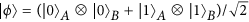, then the state vector reads:whereandfor jm {0, 1}. It is a maximally entangled state of two d-dimensional systems. In a real experiment, the entangled states that are created are not pure but are mixed states10, say, ρΦ(d), and, as will be shown presently, the purity of ρΦ(d) affects the degree of violation of the Bell inequalities.

The second step is to perform the measurements. When considering the Bell test experiment in which Alice and Bob measure the operators Aa and Bb, according to the original designations4, have the nondegenerate eigenvectorswhere (α1, α2) = (0, 1/2) and (β1, β2) = (1/4, −1/4). To take measurements from single two-state quantum particles, we rephrase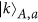andin terms of individual states aswhere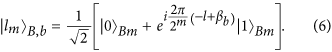Here we have used the method as the technique for decomposing the quantum Fourier transform into product representation9. For the experimental stateand ρϕm denotes the state of the mth entangled pair, the joint probabilities can be represented in terms of the probabilities of individual pairs of entangled states by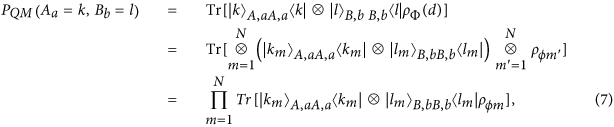which shows that the joint probability PQM(Aa = k, Bb = l) can be derived from the individual joint probabilities of obtaining the measurement outcomes km and lm on the state ρϕm. Hence, the Bell expression Id can also be determined from the outcomes of measurements of individual entangled pairs. For the case of two ensembles of perfect entangled states, the Bell inequalities can be maximally violated, that is, Id(QM) ≈ 2.970 for extremely large d-dimension4.

In the experimental demonstrations of the proposed method, we use entangled photons to construct ensembles A and B. As shown in Fig. 2, the ingredient photon pairs are generated through the type-II SPDC process and entangled at the degree of freedom of polarization21,22,23,24 which is based on the proposal Fig. 1. The polarization-entangled pair, where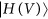represents the horizontal (vertical) polarization state. To connect with the conceptual scheme, we make a correspondence of denotations by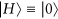and. In the experiment, the entangled pairs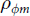consisting of the ensembles A and B are created at different times. The stability of our laser and measurement system enables entangled pairs created at different times with a large time separation have a very close fidelity without additional system alignment, which makes the experimental states approximately identical at ρϕm ≈ ρϕ for all pairs m. The tomographic diagram is depicted in Fig. 3(a)25,26,27 and the entanglement source exhibits a high quality by the state fidelity.

In addition to the Bell-CGLMP inequalities, we use the measured state fidelity FΦ(d) to extract information about the dimensionality of the entanglement between ensembles A and B. Here the entangled dimension is defined in terms of Schmidt number, S(d), of the created state ρΦ(d). For an ideal case, the Schmidt number of a perfectly prepared state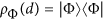is S(d) = d. We use the Schmidt number witness28,29,30 to obtain the lower bounds of the dimensionality of the entanglement. If ρΦ shows that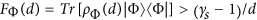then ρΦ(d) is a genuine multi-dimensional entangled state with S(d) ≥ γs where γs {2, 3, ..., d}. For a given state fidelity FΦ(d), one can find a maximum value of γs which satisfies the above condition, meaning that the maximum lower bound of S(d) can be found as well. Figure 3(b) illustrates the maximum lower bounds, SL(d), observed in our experiment.

To realize measurements in bases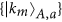[Eq. (5)] and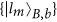[Eq. (6)], we use a half-wave plate (HWP) set at θ and quarter-wave plate (QWP) set at −π/4 to perform unitary transformations of single-photon polarization states. The angular settings of HWP for the qubits of Alice and Bob are designed as θA = −π/8 − 2π(k + αa)/4d and θB = −π/8 − 2π(−l + βb)/4d, respectively. See Methods for the complete derivation. After rotating the wave-plate sets, the states of polarizations are projected onto the base. For a given pair of operators (Aa, Bb), the total number of measurement settings of wave plates is d − 1. Although arbitrary unitary transformations of single-photon polarization states can be performed with high precision by sets of wave plates, the imperfect angle settings introduce errors that accumulate with increasing d. Such experimental imperfections become rather crucial when d is large.

In the experimental demonstration of d-dimensional nonlocality, we investigate the Bell expressions using two approaches. As quantum mechanics predicts that the Bell expression follows the definition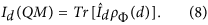whereandis the operator of Bell expression, we can obtain the values of Id(QM) either by measuring the density operator of ρΦ(d) to calculateor by performing measurements on the created states, that is, by measuring in the bases of eigenvectors of Aa and Bb [Eq. (4)] to find all of the probabilities PQM(Aa = k, Bb = l) for Id(QM).

Our experiment shows the violation of the Bell-CGLMP inequalities for systems of 16 dimensions. The Bell expressions Id(QM) are calculated by measuring all of the probabilities PQM(Aa = k, Bb = l). As seen in Fig. 4, the experimental results are strongly consistent with the theoretical predictions based on an ideal entangled stateand perfect measurements in Eq. (4). The Bell expressions measured here are strictly dependent on the accurate settings of the wave plates, as the required setting accuracy increases with d proportionally. Although our demonstration shows cases up to d = 16 only, the method can be straightforwardly extended to test Bell inequalities for systems of larger dimensionality.

We also made an estimation from the density matrices. Figure 5 illustrates that the bipartite d-dimensional system composed of polarization-entangled pairs possesses high-quality entanglement sufficient to violate Bell inequalities for d > 4000. The density matrix of the created state ρΦ(d) was experimentally measured to determine the values of Id(QM) [Eq. (8)] as the measurement of Bell expression. The degree of quantum violations depends on the purity of ρΦ(d) which is evaluated by the state fidelity FΦ(d). For the stability of our experimental conditions, the state fidelity FΦ(d) is determined by measuring Fϕ under the approximationwhich decreases with the increasing number of pairs N.

## Discussion

In this experiment, entangled photon pairs are generated to serve as a bipartite d-dimensional system to provide stronger non-locality than usual two-level cases. The concept is similar to that two entangled pairs can teleport more than one-qubit information31. For the feature that the Bell-CGLMP inequality can be tested by using entangled photon pairs created at different times, it becomes possible that one can use different experimental output states but have the same state vectors to obtain the same quantum violations. Such situations could be possible in practical quantum information tasks and their applications. For example, Alice wants to use two entangled pairs which are shared with Bob and Charlie respectively to teleport a two-qubit entangled system on her hand such that Bob and Charlie jointly share this two-qubit entanglement. In general, these two entangled pairs can be created with different entanglement sources and then used for teleportation at different times due to some technical reasons, for example, the mediate entangled pairs are generated by Bob and Charlie separately. See Fig. 6. This can be considered as an extension of the scenario used in the experiment of Zhang et al.31 where the two entangled pairs are created at the same time. While the entangled pairs are created at different times by using different sources, the target two-qubit entangled state is enabled to be teleported in principle. Our scenario can concretely reveal the quantum nonlocality that powers this two-qubit teleportation by violating the Bell-CGLMP inequality.

Extending this idea, a multi-partite multi-dimensional system will be implemented in the future work by genuine multi-partite entanglement of two-dimensional systems. With this approach, it would be possible to investigate generic quantum nonlocality by systematically implementing Bell test experiments for the multi-partite multi-dimensional systems5. There have been extensive investigations of multi-partite entanglement, both from the fundamental and practical points of view. In particular, a genuine eight-photon polarization-entangled state has recently been experimentally generated using the SPDC process32. Hence, our novel scheme holds high promise for testing generic Bell inequalities for the eight-partite high-dimensional systems using a state-of-the-art eight-photon entanglement source. In addition to entangled photons, one can directly apply our idea to other quantum systems where the states are able to be coherently manipulated and locally measured. Such kind of Bell test experiments could be realized in systems of multi-partite entangled ions in the Greenberger-Horne-Zeilinger (GHZ) state33. The systems of nanomechanical resonators34 and Josephson circuits35,36 also provide these suitable conditions to implement our scheme. Furthermore, it is interesting to investigate violations of CGLMP-Bell inequality with multi-dimensional entanglement composed of intra- and inter-pair entangled states37. While the devised scenario still requires a rigorous theory showing its general utility in quantum applications such as quantum information processing, it provides a novel way to consider all the related information tasks based on the generic Bell test experiments.

Finally, we remark three points on the present experimental violations of Bell-CGLMP inequalities. First, while the ensemble of photon pairs is mathematically equivalent to a multi-dimensional entangled state, each Bell pair is generated and immediately afterwards already detected and then such a multi-dimensional entangled state is not generated at any point of time. Here, our aim is to demonstrate that the test of Bell-CGLMP inequalities can be shown by sub-systems even though the particles never co-existed. Second, using the independently generated Bell pairs to realize a quantum information protocol, such as quantum teleportation introduced above (Fig. 6), can use quantum memory38,39 for state storage so that they can co-exist before the subsequent required measurements. This is different from the implementation which utilizes multi-dimensional states of genuinely single quantum systems16. Compared with the teleportation scheme without memory, Fig. 6, the states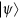can be teleported to Bob and Charlie at the same time with the support of quantum memory, which gives higher flexibility for a subsequent quantum information task. Finally, in addition to the present experimental demonstration using SPDC source, there exists other potential methods to create ensembles of entangled photons to show the general utility of our idea in the Bell test experiments on multi-dimensional systems. For instance, one can use hyperentangled states to investigate quantum violations of Bell-CGLMP inequalities. As shown in the experiments on such entanglement40,41, the two-photon four-qubit state entangled both in polarization and spatial modes has been created as, whereand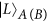represent two orthogonal states with the spatial modes RA(B) and LA(B). This hyperentangled state is equivalent to a maximally entangled state of two four-dimensional systems [See Eq. (3)] and then suitable for implementing our scheme.

In conclusion, we have demonstrated the violation of the Bell-CGLMP inequalities for systems of extremely large dimensions. These demonstrations are based on bipartite d-dimensional systems composed of polarization-entangled photon pairs. Using the well-known technique of type-II SPDC for polarization entanglement, which is robust and stable and needs only modest experimental effort using standard technical devices such as wave-plate sets and SPCMs, the scheme provides a way to experimentally observe d-dimensional quantum nonlocality up to d = 16 by performing measurements of the Bell expressions Id. An estimation of the quantum nonlocality also shows up to d = 4096 by measuring the density matrix of the entangled state.

## Methods

### Measurements on single entangled photon pairs

To realize the measurements according to Eq. (7), the probabilities are determined by firstly performing local transformation on photons. We use HWP and QWP to perform the required rotations of polarization states. See Fig. 2. The HWP and QWP can be described by the following transformations, respectively27:wheredenotes the photon state with horizontal (vertical) polarization. Hence, with combination of both wave plates, the local transformation for polarization state is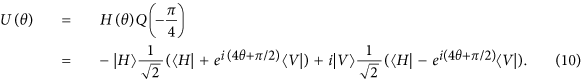To implement measurements onto the state setsand, the angular settings of HWP for the qubits of Alice and Bob are designed as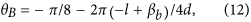respectively. After such transformations, the statesandwill become horizontal-polarization statesand. Thus determining the joint probability of measuringandfor P(Aa, Bb) is equivalent to measuring the joint probability P(H, H) on the m-th entangled photon pair [UA) UB)]ρϕm[UA) UB)].

How to cite this article: Lo, H.-P. et al. Experimental violation of Bell inequalities for multi-dimensional systems. Sci. Rep. 6, 22088; doi: 10.1038/srep22088 (2016).

## References

1. Bell, J. S. On the Einstein Podolsky Rosen paradox. Physics (Long Island City, N.Y.) 1, 195–200 (1964).

2. Clauser, J., Horne, M., Shimony, A. & Holt, R. Proposed Experiment to Test Local Hidden-Variable Theories. Phys Rev Lett 23, 880–884 (1969).

3. Kaszlikowski, D., Gnacinski, P., Zukowski, M., Miklaszewski, W. & Zeilinger, A. Violations of Local Realism by Two Entangled N-Dimansional Systems Are Stronger than for Two Qubits. Phys Rev Lett 85, 4418–4421 (2000).

4. Collins, D., Gisin, N., Linden, N., Massar, S. & Popescu, S. Bell Inequalities for Arbitrarily High-Dimensional Systems. Phys Rev Lett 88, 040404 (2002).

5. Son, W., Lee, J. & Kim, M. S. Generic Bell Inequalities for Multipartite Arbitrary Dimensional Systems. Phys. Rev. Lett. 96, 060406 (2006).

6. Einstein, A., Podolsky, B. & Rosen, N. Can Quantum-Mechanical Description of Physical Reality Be Considered Complete? Phys. Rev. 47, 777–780 (1935).

7. Schrödinger, E. Die gegenwartige situation in der quantenmechanik. Naturwissenschaften 23, 807–812; 823–828; 844–849 (1935).

8. Bell, J. S. Speakable and Unspeakable in Quantum Mechanics. Cambridge University Press: Cambridge, UK (1987).

9. Nielsen, M. A. & Chuang, I. L. Quantum Computation and Quantum Information. Cambridge University Press: Cambridge, UK (2000).

10. Pan, J.-W., Chen, Z.-B., Zukowski, M., Weinfurter, H. & Zeilinger, A. Multiphoton entanglement and interferometry. Rev Mod Phys 84, 777–838 (2012).

11. Vaziri, A., Weihs, G. & Zeilinger, A. Experimental Two-Photon, Three-Dimensional Entanglement for Quantum Communication. Phys Rev Lett 89, 240401 (2002).

12. Gröblacher, S., Jennewein, T., Vaziri, A., Weihs, G. & Zeilinger, A. Experimental quantum cryptography with qutrits. New J Phys 8, 75 (2006).

13. Zhou, D. L., Zeng, B., Xu, Z. & Sun, C. P. Quantum computation based on d-level cluster state. Phys Rev A 68, 062303 (2003).

14. Looi, S. Y., Yu, L., Gheorghiu, V. & Griffiths, R. B. Quantum-error-correcting codes using qudit graph states. Phys Rev A 78, 042303 (2008).

15. Lloyd, S. Enhanced Sensitivity of Photodetection via Quantum Illumination. Science 321, 1463–1465 (2008).

16. Dada, A. C., Leach, J., Buller, G. S., Padgett, M. J. & Andersson, E. Experimental high-dimensional two-photon entanglement and violations of generalized Bell inequalities. Nat Phys 7, 677–680 (2011).

17. Mair, A., Vaziri, A., Weihs, G. & Zeilinger, A. Entanglement of the orbital angular momentum states of photons. Nature (London) 412, 313–316 (2001).

18. Allen, L., Beijersbergen, M. W., Spreeuw, R. J. C. & Woerdman, J. P. Orbital angular momentum of light and the transformation of Laguerre-Gaussian laser modes. Phys Rev A 45, 8185–8189 (1992).

19. Romero, J., Giovannini, D., Franke-Arnold, S., Barnett, S. M. & Padgett, M. J. Increasing the dimension in high-dimensional two-photon orbital angular momentum entanglement. Phys Rev A 86, 012334 (2012).

20. Krenn, M. et al. A. Generation and confirmation of a (100 × 100)-dimensional entangled quantum system. PNAS 111, 6243–6247 (2014).

21. Kwiat, P. G. et al. New high-intensity source of polarization-entangled photon pairs. Phys Rev Lett 75, 4337–4341 (1995).

22. Lo, H. P., Yabushita, A., Luo, C. W., Chen, P. & Kobayashi, T. Beamlike photon-pair generation for two-photon interference and polarization entanglement. Phys Rev A 83, 022313 (2011).

23. Lo, H. P., Yabushita, A., Luo, C. W., Chen, P. & Kobayashi, T. Beamlike photon pairs entangled by a 2 × 2 fiber. Phys Rev A 84, 022301 (2011).

24. Lo, H. P., Yabushita, A. & Kobayashi, T. Photon doughnut-shaped pair for easy production of entangled photon pairs. Phys Rev A 91, 062327 (2015).

25. Vogel, K. & Risken, H. Determination of quasiprobability distributions in terms of probability distributions for the rotated quadrature phase. Phys Rev A 40, 2847–2849 (1989).

26. Hradil, Z., Řeháček, J., Fiurášek, J. & Ježek, M. Maximum-Likelihood Methods in Quantum Mechanics. Lecture Notes in Physics 649, 59–112 (2004).

27. James, D. F. V., Kwiat, P. G., Munro, W. J. & White, A. G. Measurement of qubits. Phys Rev A 64, 052312 (2001).

28. Terha, B. M. & Horodecki, P. Schmidt number for density matrices. Phys Rev A 61, 040301(R) (2000).

29. Li, C.-M., Chen, K., Reingruber, A., Chen, Y.-N. & Pan, J.-W. Verifying Genuine High-Order Entanglement. Phys Rev Lett 105, 210504 (2010).

30. Sanpera, A. & Bruß, D. Lewenstein M. Schmidt-number witnesses and bound entanglement. Phys Rev A 63, 050301(R) (2001).

31. Zhang, Q. et al. Experimental quantum teleportation of a two-qubit composite system. Nat Phys 2, 678–682 (2006).

32. Yao, X.-C. et al. Observation of eight-photon entanglement. Nat Photonics 6, 225–228 (2012).

33. Monz, T. et al. 14-Qubit Entanglement: Creation and Coherence. Phys Rev Lett 106, 130506 (2011).

34. Johansson, J. R., Lambert, N., Mahboob, I., Yamaguchi, H. & Nori, F. Entangled-state generation and Bell inequality violations in nanomechanical resonators. Phys Rev B 90, 174307 (2014).

35. Wei, L. F., Liu, Y. X. & Nori, F. Testing Bell’s inequality in constantly coupled Josephson circuits by effective single-qubit operations. Phys Rev B 72, 104516 (2005).

36. Wei, L. F., Liu, Y. X., Storcz, M. J. & Nori, F. Macroscopic Einstein-Podolsky-Rosen pairs in superconducting circuits. Phys Rev A 73, 052307 (2006).

37. Ashhab, S., Maruyama, K., Brukner, C. & Nori, F. Bell’s experiment with intra- and inter-pair entanglement: Single-particle mode entanglement as a case study. Phys Rev A 80, 062106 (2009).

38. Chen, Y.-A. et al. Memory-built-in quantum teleportation with photonic and atomic qubits. Nat Phys 4, 103 (2008).

39. Yuan, Z.-S. et al. Entangled photons and quantum communication. Phys Rep 497, 1 (2010).

40. Yang, T. et al. All-versus-nothing violation of local realism by two-photon, four-dimensional entanglement. Phys Rev Lett 95, 240406 (2005).

41. Chen, K. et al. Experimental realization of one-way quantum computing with two-photon four-qubit cluster states. Phys Rev Lett 99, 120503 (2007).

## Acknowledgements

This work was supported by the Ministry of Science and Technology, Taiwan, Republic of China (Contract No. 101-2112-M-009-002-MY2, 103-2112-M-009-008, 101-2112-M-006-016-MY3, 101-2628-M-006-003-MY3, 101-2112-M-009-016-MY2, 103-2119-M-009-004-MY3, 103-2628-M-009-002-MY3, 103-2923-M-009-001-MY3, 103-2112-M-006-017-MY4, 104-2112-M-009-001-MY2 and 104-2112-M-006-016-MY3) and the Grant MOE ATU Program at NCTU.

## Author information

Authors

### Contributions

H.P.L., A.Y., C.W.L. and T.K. carried out the experiment; C.-M.L. devised the theoretical and experimental scheme and Y.-N.C. provided the theoretical analysis; H.P.L., A.Y., C.W.L. and T.K. conceived the experiment and analysed the data; and all authors co-wrote the paper.

## Ethics declarations

### Competing interests

The authors declare no competing financial interests.

## Rights and permissions

Reprints and Permissions

• ### From contextuality of a single photon to realism of an electromagnetic wave

• Marcin Markiewicz
• , Dagomir Kaszlikowski
• , Paweł Kurzyński
•  & Antoni Wójcik

npj Quantum Information (2019)

• ### Bell’s inequality tests via correlated diffraction of high-dimensional position-entangled two-photon states

• Wei Li
•  & Shengmei Zhao

Scientific Reports (2018)